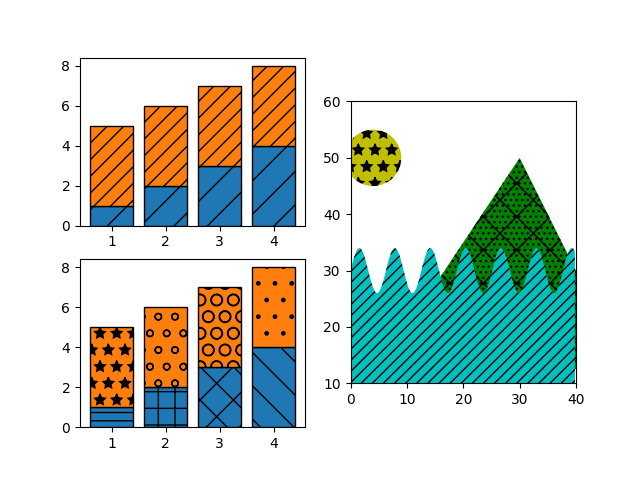# Hatch demo¶

Hatches can be added to most polygons in Matplotlib, including bar, fill_between, contourf, and children of Polygon. They are currently supported in the PS, PDF, SVG, OSX, and Agg backends. The WX and Cairo backends do not currently support hatching.

See also Contourf Hatching for an example using contourf, and Hatch style reference for swatches of the existing hatches.

import numpy as np
import matplotlib.pyplot as plt
from matplotlib.patches import Ellipse, Polygon

x = np.arange(1, 5)
y1 = np.arange(1, 5)
y2 = np.ones(y1.shape) * 4

fig = plt.figure()
axs = fig.subplot_mosaic([['bar1', 'patches'], ['bar2', 'patches']])

axs['bar1'].bar(x, y1, edgecolor='black', hatch="/")
axs['bar1'].bar(x, y2, bottom=y1, edgecolor='black', hatch='//')

axs['bar2'].bar(x, y1, edgecolor='black', hatch=['--', '+', 'x', '\\'])
axs['bar2'].bar(x, y2, bottom=y1, edgecolor='black',
hatch=['*', 'o', 'O', '.'])

x = np.arange(0, 40, 0.2)
axs['patches'].fill_between(x, np.sin(x) * 4 + 30, y2=0,
hatch='///', zorder=2, fc='c')
axs['patches'].add_patch(Ellipse((4, 50), 10, 10, fill=True,
hatch='*', facecolor='y'))
axs['patches'].add_patch(Polygon([(10, 20), (30, 50), (50, 10)],
hatch='\\/...', facecolor='g'))
axs['patches'].set_xlim([0, 40])
axs['patches'].set_ylim([10, 60])
axs['patches'].set_aspect(1)
plt.show()References

The use of the following functions, methods, classes and modules is shown in this example:

Keywords: matplotlib code example, codex, python plot, pyplot Gallery generated by Sphinx-Gallery